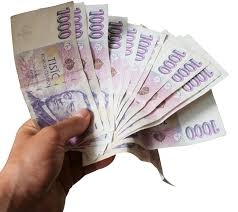# Refreshments 7371

Milan got a certain amount of money for the trip. 1/6 missed postcards, 1/4 on refreshments. He bought his brothers a gift for 140 Euros. How many euros did he receive?

n =  240

### Step-by-step explanation:

n/6 + n/4 + 140 =n

7n = 1680

n = 1680/7 = 240

n = 240

Our simple equation calculator calculates it.Did you find an error or inaccuracy? Feel free to write us. Thank you!

Tips for related online calculators
Need help calculating sum, simplifying, or multiplying fractions? Try our fraction calculator.
Do you have a linear equation or system of equations and looking for its solution? Or do you have a quadratic equation?